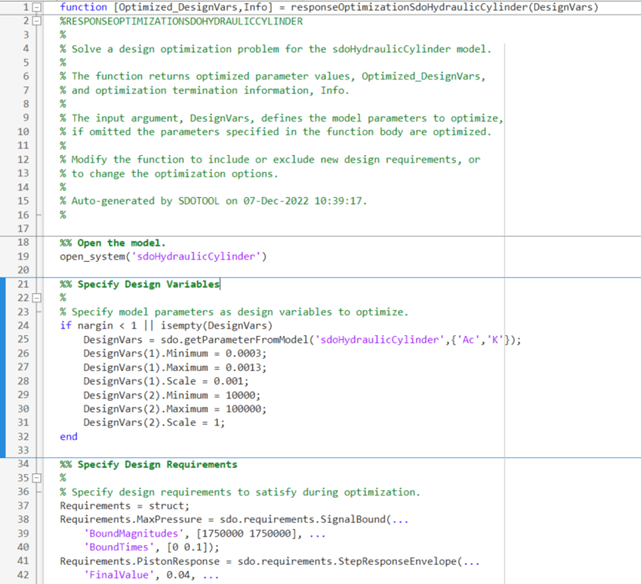Generate MATLAB Code for Design Optimization Problems (GUI)

This example shows how to automatically generate a MATLAB® function to solve a Design Optimization problem. You use the Response Optimizer to define an optimization problem for a hydraulic cylinder design and generate MATLAB code to solve this optimization problem.

Hydraulic Cylinder Design Problem

The Design Optimization to Meet a Custom Objective (GUI) example shows how to use the Response Optimizer to optimize a cylinder design. In this example we load a pre-configured Response Optimizer session based on that example.

Use the following command to open the Response Optimizer

sdotool(SDOSessionData)

Generate MATLAB Code

From the Optimize list, select Generate MATLAB Code.The generated code is added to the MATLAB editor as an unsaved MATLAB function.Examine the generated code. Significant code portions are:

• Specify Design Variables - Definition of the model parameters being optimized.

• Specify Design Requirements - Definition of the design requirements.

• Create Optimization Objective Function - Creation of an anonymous function that calls the subfunction sdoHydraulicCylinder_optFcn, which evaluates the cylinder design. sdo.optimize calls the anonymous function at each iteration.

• Evaluate custom parameter requirement functions - Evaluates the custom requirement, MinimizeAC, that uses the sdoHydraulicCylinder_customObjective function.

• Optimize the Design - Optimization using the sdo.optimize command.

Select Save from the MATLAB editor to save the generated function.

Run Generated Code

Run the generated function.The first output argument, pOpt, contains the optimized parameter values and the second output argument, optInfo, contains optimization information.

Modify the Generated Code

You can:

• Modify the generated sdo_sdoHydraulicCylinder function to include or exclude new design requirements or change the optimization options.

• Call the generated sdo_sdoHydraulicCylinder function with a different set of parameters to optimize.

For details on how to write an objective/constraint function to use with the sdo.optimize command, type help sdoExampleCostFunction at the MATLAB command prompt.

Close the model.